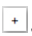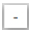# Problem: Assign formal charges to each atom in the O3 molecule shown here. Be sure to click the  or  button before clicking on the molecule.Click the  or  button to select it, then click on an atom, and then type the desired charge.To determine the formal charges of the atoms in a molecule, we must assign each electron to a particular atom. Then, we compare the number of electrons assigned to each atom to the expected valence of that element.All lone pairs of electrons should be assigned to the atom on which they are found; bonding pairs of electrons should be divided equally between the atoms in the bond. The formal charge is calculated by subtracting the number of electrons assigned to the atom from the number of valence electrons in the free atom.FC = (valence e- in free atom) - [nonbonding e- shown + 12bonding e- shown]For example, in C≡O, each atom is assigned five electrons (two nonbonding from the atom's lone pair and three bonding from half of the triple bond). Since the expected valence of carbon is four, its formal charge is (4 valence) - (5 assigned) = -1. Since the expected valence of oxygen is six, its formal charge is (6 valence) - (5 assigned) = +1For a neutral molecule such as CO, the sum of the formal charges is zero. For a polyatomic ion, the sum of the formal charges is equal to the overall charge on the ion.

###### FREE Expert Solution
95% (226 ratings)View Complete Written Solution
###### Problem Details

Assign formal charges to each atom in the O3 molecule shown here. Be sure to click theorbutton before clicking on the molecule.

Click theorbutton to select it, then click on an atom, and then type the desired charge.

To determine the formal charges of the atoms in a molecule, we must assign each electron to a particular atom. Then, we compare the number of electrons assigned to each atom to the expected valence of that element.

All lone pairs of electrons should be assigned to the atom on which they are found; bonding pairs of electrons should be divided equally between the atoms in the bond. The formal charge is calculated by subtracting the number of electrons assigned to the atom from the number of valence electrons in the free atom.

For example, in C≡O, each atom is assigned five electrons (two nonbonding from the atom's lone pair and three bonding from half of the triple bond). Since the expected valence of carbon is four, its formal charge is (4 valence) - (5 assigned) = -1. Since the expected valence of oxygen is six, its formal charge is (6 valence) - (5 assigned) = +1

For a neutral molecule such as CO, the sum of the formal charges is zero. For a polyatomic ion, the sum of the formal charges is equal to the overall charge on the ion.